International
Tables for
Crystallography
Volume B
Reciprocal space
Edited by U. Shmueli

International Tables for Crystallography (2006). Vol. B, ch. 5.1, pp. 548-549   | 1 | 2 |

## Section 5.1.8.2. Borrmann triangle

A. Authiera*

aLaboratoire de Minéralogie-Cristallographie, Université P. et M. Curie, 4 Place Jussieu, F-75252 Paris CEDEX 05, France
Correspondence e-mail: authier@lmcp.jussieu.fr

#### 5.1.8.2. Borrmann triangle

| top | pdf |

The first property of the Borrmann triangle is that the angular density of the wavefield paths is inversely proportional to the curvature of the dispersion surface around their tie points. Let us consider an incident wavepacket of angular width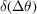. It will generate a packet of wavefields propagating within the Borrmann triangle. The angular width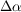(Fig. 5.1.8.2) between the paths of the corresponding wavefields is related to the radius of curvatureof the dispersion surface by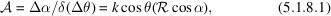where α is the angle between the wavefield path and the lattice planes [equation (5.1.2.26)] andis called the amplification ratio. In the middle of the reflecting domain, the radius of curvature of the dispersion surface is very much shorter than its value, k, far from it (about 104 times shorter) and the amplification ratio is therefore very large. As a consequence, the energy of a wavepacket of widthin reciprocal space is spread in direct space over an anglegiven by (5.1.8.1). The intensity distribution on the exit surface BC (Fig. 5.1.8.1a) is therefore proportional to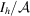. It is represented in Fig. 5.1.8.3for several values of the absorption coefficient:

• (i) Small values of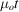(less than 2 or 3) (Fig. 5.1.8.3a). The intensity distribution presents a wide minimum in the centre where the density of wavefields is small and increases very sharply at the edges where the density of wavefields is large, although it is the reverse for the reflecting power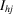. This effect, called the margin effect, was predicted qualitatively by Borrmann (1959)and von Laue (1960), demonstrated experimentally by Kato & Lang (1959), and calculated by Kato (1960).Figure 5.1.8.2 | top | pdf |Packet of wavefields of divergence Δα excited in the crystal by an incident wavepacket of angular width. (a) Direct space; (b) reciprocal space.Figure 5.1.8.3 | top | pdf |Intensity distribution along the base of the Borrmann triangle. y is a normalized coordinate along BC. (a) Small values of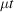. The interference (spherical-wave Pendellösung) between branch 1 and branch 2 is neglected. (b) Large values of.
• (ii) Large values of(of the order of 6 or more) (Fig. 5.1.8.3b). The predominant factor is now anomalous absorption. The wavefields propagating along the edges of the Borrmann triangle undergo normal absorption, while those propagating parallel to the lattice planes (or nearly parallel) correspond to tie points in the centre of the dispersion surface and undergo anomalously low absorption. The intensity distribution now has a maximum in the centre. For values oflarger than 10 or so, practically only the wavefields propagating parallel to the lattice planes go through the crystal, which acts as a wave guide: this is the Borrmann effect.

### References

Borrmann, G. (1959). Röntgenwellenfelder. Beit. Phys. Chem. 20 Jahrhunderts, pp. 262–282. Braunschweig: Vieweg und Sohn.Google Scholar
Kato, N. (1960). The energy flow of X-rays in an ideally perfect crystal: comparison between theory and experiments. Acta Cryst. 13, 349–356.Google Scholar
Kato, N. & Lang, A. R. (1959). A study of Pendellösung fringes in X-ray diffraction. Acta Cryst. 12, 787–794.Google Scholar
Laue, M. von (1960). Röntgenstrahl-Interferenzen. Frankfurt am Main: Akademische Verlagsgesellschaft.Google Scholar